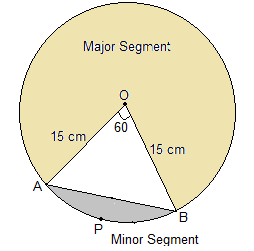Newbie

# A chord of a circle of radius 15 cm subtends an angle of 60° at the centre. Find the areas of the corresponding minor and major segments of the circle. (Use π = 3.14 and √3 = 1.73) Q.6

• 0

Find the best solution of ncert class 10 of areas related to circles of exercise 12.2 question no.6 Give me the best and easiest solution of this question its very tricky question please give me the simplest solution. A chord of a circle of radius 15 cm subtends an angle of 60° at the centre. Find the areas of the corresponding minor and major segments of the circle. (Use π = 3.14 and √3 = 1.73)

Share

1. on:Given,

Radius = 15 cm

θ = 60°

So,

Area of sector OAPB = (60°/360°)×πrcm2

= 225/6 πcm2

Now, ΔAOB is equilateral as two sides are the radii of the circle and hence equal and one angle is 60°

So, Area of ΔAOB = (√3/4) ×a2

Or, (√3/4) ×152

∴ Area of ΔAOB = 97.31 cm2

Now, area of minor segment APB = Area of OAPB – Area of ΔAOB

Or, area of minor segment APB = ((225/6)π – 97.31) cm= 20.43 cm2

And,

Area of major segment = Area of circle – Area of segment APB

Or, area of major segment = (π×152) – 20.4 = 686.06 cm2

• 0